HLIBpro  2.3.1
Visualisation

In contrast to storage, visualisation will print certain aspects of the corresponding data, e.g. block structure of a block cluster tree or the block wise rank in case of 𝓗-matrices. 𝓗𝖫𝖨𝖡𝗉𝗋𝗈 uses PostScript, PDF, VRML and VTK for visualisation, albeit the last two are reserved for 3D objects, e.g. coordinates or grids.

Remarks
All visualisation classes will automatically add a corresponding filename suffix, e.g. ".eps" in case of (encapsulated) PostScript, if no suffix is present.

# Coordinate Visualisation

Coordinate sets can be visualised in PostScript, VRML or VTK file format, implemented in TPSCoordVis, TVRMLCoordVis and TVTKCoordVis respectively. Each of these visualisation classes provide the basic function

void print ( const TCoordinate * coord,
const String & filename ) const;

for printing the coordinates to file filename. Furthermore, the various classes also provide special function to visualise clusters or block clusters using the coordinate information in coord.

In addition to this, a visualisation function is provided which enables to label individual coordinates:

void print ( const TCoordinate * coord,
const std::vector< uint > & label,
const String & filename ) const;

Here, label is at least of size coord and contains in `label`[i] a label for the coordinate `i`. All coordinates with the same label will be drawn with the same colour. Currently, at most 8 different colours are supported. Please note, that a label of `0` is considered as a neutral label, i.e. drawn with a neutral colour.

An application of this is the visualisation of block clusters:

TBlockCluster * bc = ... // some block cluster
vector< uint > label( n, 0 ); // by default: all indices label neutral
// label row and column cluster indices differently
for ( idx_t i = bc->rowcl()->first(); i <= bc->rowcl()->last(); ++i ) label[i] = 1;
for ( idx_t i = bc->colcl()->first(); i <= bc->colcl()->last(); ++i ) label[i] = 2;
TVTKCoordVis cvis;
cvis( coord.get(), label, "label_coord" );

This will produce the red and green coloured coordinates in the following figure: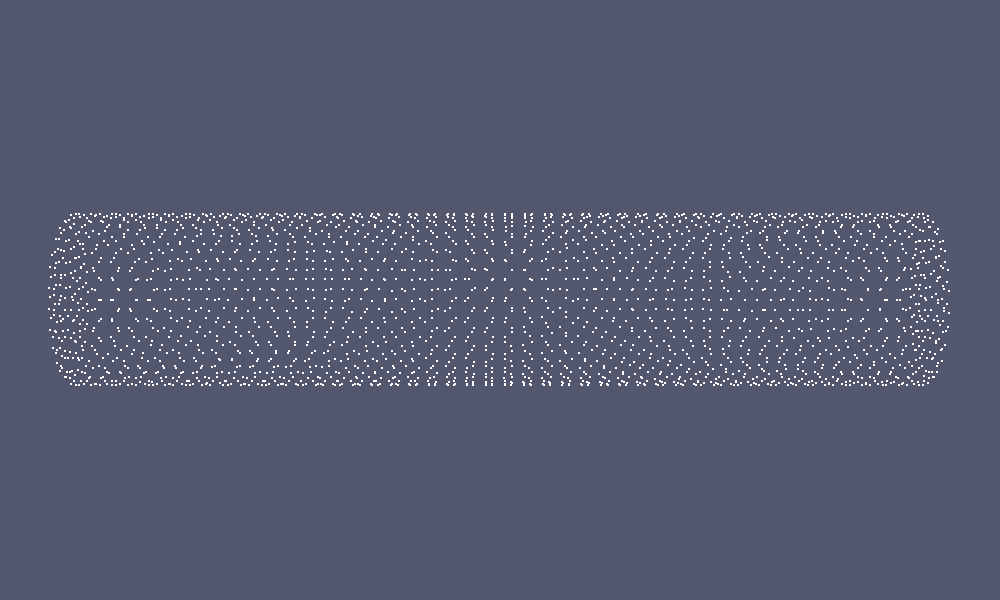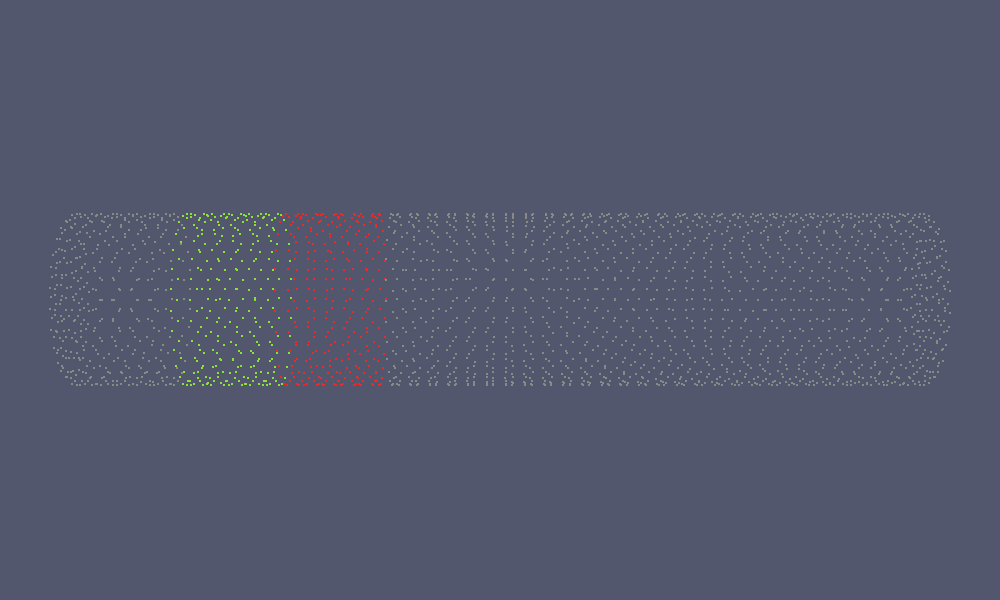Coordinate Set Labeled Coordinate Set

# Matrix Visualisation

Matrix visualisation in 𝓗𝖫𝖨𝖡𝗉𝗋𝗈 is solely implemented in TPSMatrixVis, e.g. in PostScript format. The output can be changed via several options to show different aspects of the matrix, e.g. the block structure, non-zero pattern, local ranks or singular value distribution. The basic output function of TPSMatrixVis is

void print ( const TMatrix * A, const String & filename ) const;

which will print the matrix A into the file filename.

Options are also set via functions, where for most functions the only argument is of boolean type and either turns the corresponding feature on or off:

Function Description
`structure(b)`

Print the block structure of the matrix. This is the default setting.

`nonempty(b)`

Only print nonempty blocks.

`entries(b)`

Print matrix coefficients

`pattern(b)`

Print sparsity pattern of matrix.

`sparse_pattern(b)`

Print sparsity pattern of matrix only for blocks of type TSparseMatrix.

`svd(b)`

Print singular values for each low-rank and dense block of the matrix.

`svd_ref(max,min)`

Define scaling interval for block-wise singular values, e.g. maximal and miminal values are assumed to be max and min. If max/min 0, the largest and smallest local singular values per block are used instead. A good choice for max is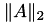. min can be ommited in which case the machine precision is used.

`rank_col(b)`

Colour the low-rank blocks according to local rank vs. full rank.

`max_size_ratio(r)`

Set maximal allowed ratio of local block size (no. of rows or columns) compared to matrix size, e.g. block with a larger ratio (and hence, smaller size), will not be printed (for very large matrices this limits file size).

The option functions can also be concatenated:

TMatrix * A = ...; // define matrix
TPSMatrixVis mvis;
mvis.svd( true ).svd_ref( norm_2( A ) );
mvis.print( A, "A.ps" );

The following images show different output settings of the same matrix: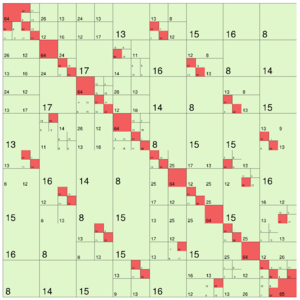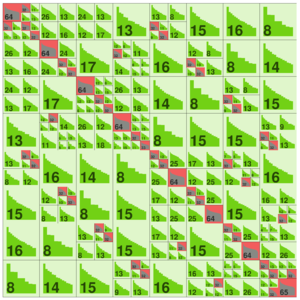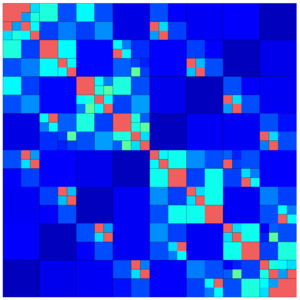default output (`structure` = true) `svd` = true `rankcol` = true, `structure` = false

Here, each type of matrix block is printed with a distinct colour (assuming `rank_col` = false):

•       : NULL or empty blocks (on case of `nonempty` = true)
•       : dense
•       : low-rank
•       : uniform
•       : sparse

# Grid Visualisation

Grids may be printed in either PostScript, VRML or VTK format, implemented in TPSGridVis, TVRMLGridVis and TVTKGridVis respectively. Each of these visualisation classes provide the basic function

void print ( const TGrid * grid,
const String & filename ) const;

to print the given grid to a file. Beside this, it is also possible to print a given grid function defined by a real, scalar vector over a function space using:

void print ( const TGrid * grid,
const TFnSpace * fnspace,
const TVector * vec,
const String & filename ) const;

The function is here drawn using a colour map corresponding to the function values at the triangle points.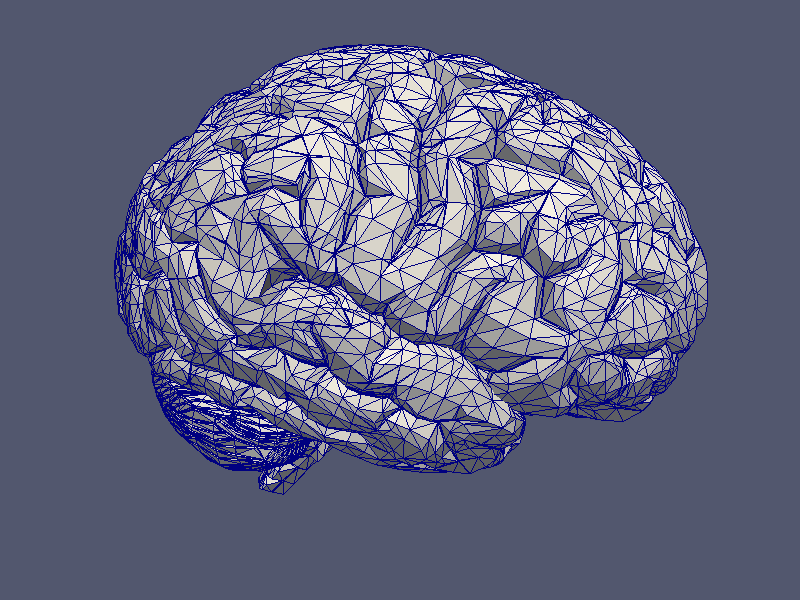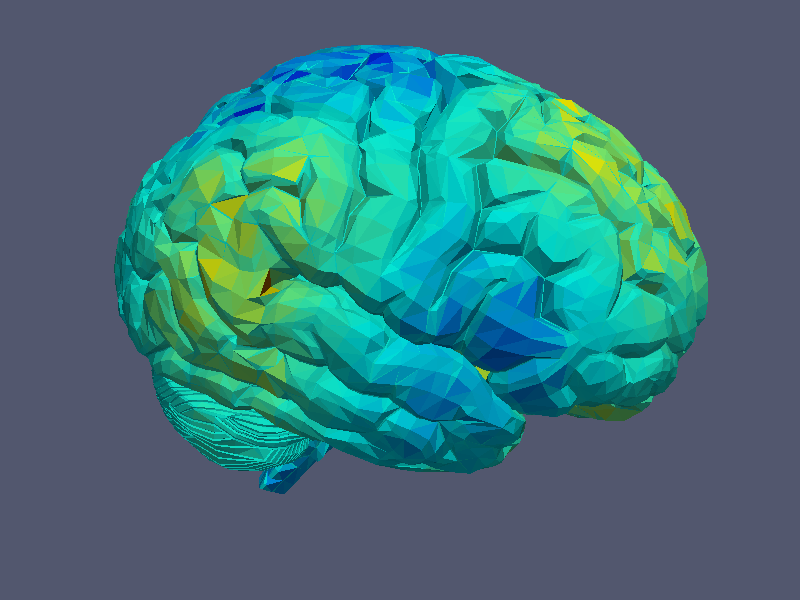Grid Visualisation Grid Function Visualisation
Remarks
For the original grid file, please see http://gfx.cs.princeton.edu/proj/sugcon/models/.

You may choose a different colourmap with the function `set_colourmap`, which supports the following arguments: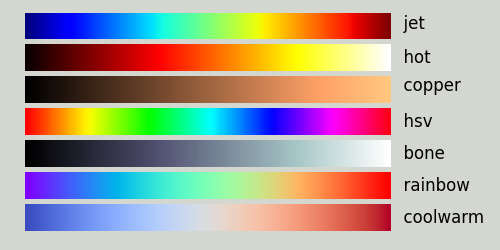Supported Colour Maps

Furthermore, by default the interval for the printed values is determined by the smallest and the largest value in the provided vector. You may override this with the function `set_func_value_interval`.

Remarks
Plase note, that the vector has to be real valued, i.e. complex valued vectors are not supported.